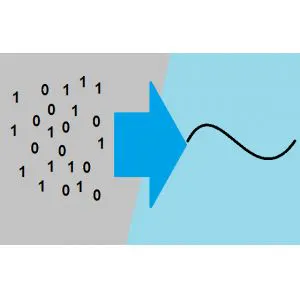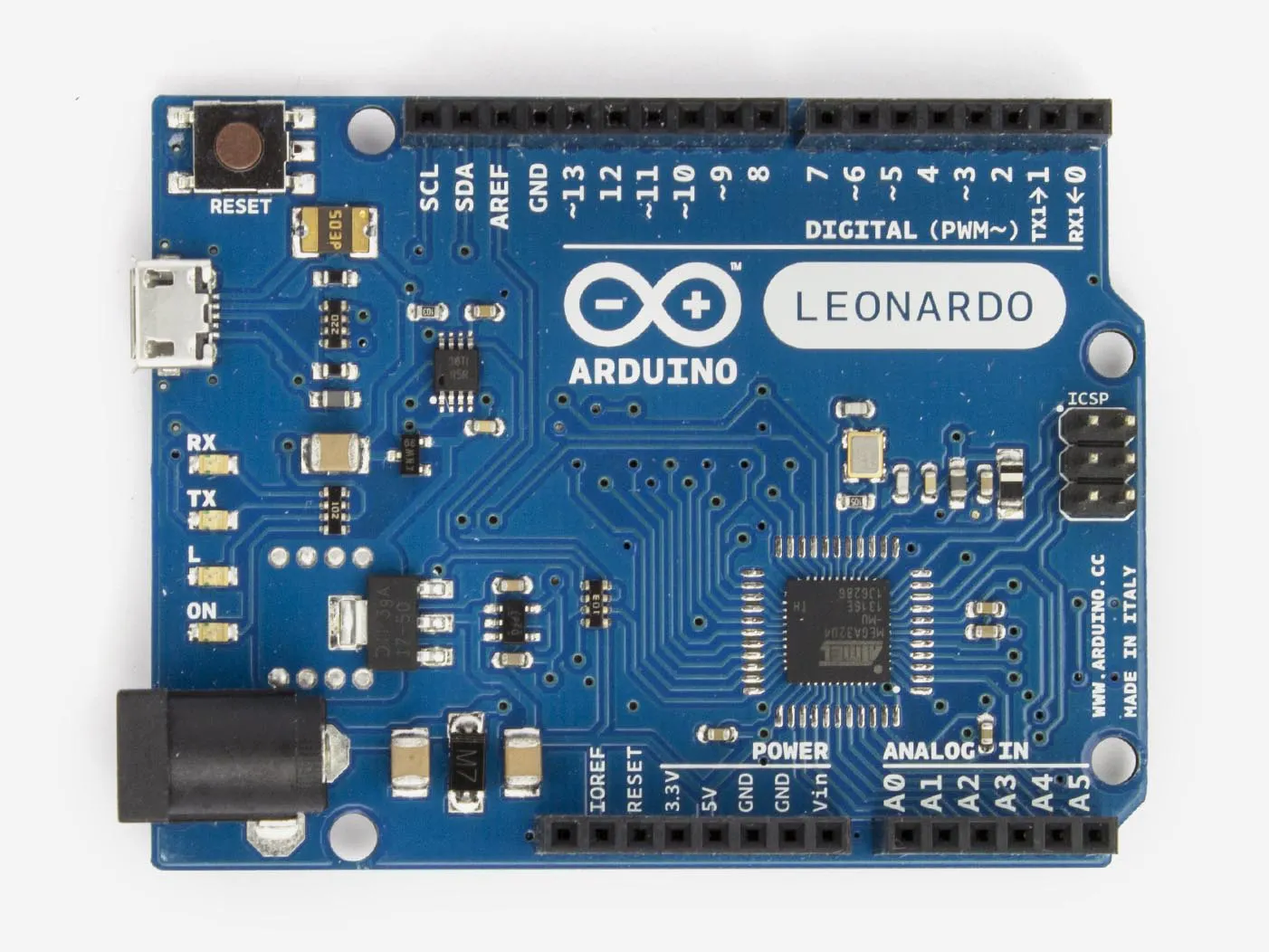Project showcaseA DAC is a circuit that allows you to translate numeric values into analog signals.

• 102,340 views
• 13 respects

## Components and suppliesArduino Leonardo
×1
×1
 TL2451
×1
 Capacitor 22 µF
×1
 Resistor 3.3 KOhm
×1

### 1. Introduction

A DAC is a circuit that allows you to translate numeric values into analog signals, so you can have output voltages variable from 0 to 5V by setting only a variable. If you want to do this with an Arduino different from the Due you can't without using an external chip.

With few components you can build a DAC circuit that will do a nice job in low-speed and low-precision applications. This will work as DAC because you can have output voltages between 0V and 5V by only modifying a variable between 0 and 255.

To build it you will need:

• TLV2451 Operational Amplifier (you can use almost every operational amplifier, just remember to check that is rail-to-rail).
• 22 uF capacitor
• 3.3 kOhm resistor.

### 2. The idea: PWM and Duty Cycle

Every Arduino has PWM output capabilities, it can output a square waveform with variable duty-cycle through the `analogWrite` function.

The duty-cycle is the ratio of the ON time and the total period of the wave. The ON time is the amount of time in which the wave stays at 5V and the total period is the sum of the time while it stays at 5V and 0V time.

When you use the `AnalogWrite `function you set the output duty cycle that may vary between 0% and 100% and you choose these values with the second parameter you pass to the function ( 0÷255).

DC = ON TIME/(OFF TIME + ON TIME)

### 3. The idea: Duty cycle and mean Value

The mean value of a wave is the average of the voltage provided from the output over a period time of the wave.

The square waveform with amplitude 0V-5V has a mean value that is equal to the max amplitude (5V) multiplied for the duty-cycle. This means that you can change the mean value of the PWM output by using the `analogWrite` function.

For example if you set the duty cycle at 0% (`analogWrite(pin,0)`) you will have a mean value of 0V, if you set it at 50% (`analogWrite(pin,127)`) you will  have a 2.5V average value, and 5V with 100%).

### 4. The idea: Mean value and filter

Now that you know how to modify the mean value of the wave, you need something that allows you to use it.

According to mathematicians every signal is formed by the sum of many simple signals that differ each other by the  frequency and amplitude. The mean value of the wave is one of these simple signals, it is exactly the value at zero frequency or the well known DC value.

This DC value is what you need to have a DC current output that is the desired output of your DAC. You can extract the DC value of the signal by using a low-pass filter that allows only the DC signal to pass and blocks nearly all others signals. You can't get high precision filter with these components but is good enough for simple applications.

### 5. Build the filter circuit

This circuit filters unwanted frequencies and is very simple to build.

The output is the orange wire and it will provide the DC output you want.

### 6. Using the circuit

To use the circuit you only have to connect the input on a digital pin with PWM, and use the `analogWrite` function on your sketch. The code shown below will output a square wave of 50% duty cycle on pin 5 and the DAC will output around 2.5V.

The image shows the output of the Arduino and the filtered output of the DAC.

``````void setup() {
// initialize the digital pin as an output.
pinMode(5, OUTPUT);
}
void loop() {
analogWrite(5,127);  //Set the PWM at 50 % on digital pin 5
}
``````

## Code

##### Code snippet #1Arduino
```void setup() {
// initialize the digital pin as an output.
pinMode(5, OUTPUT);
}

void loop() {
analogWrite(5,127);  //Set the PWM at 50 % on digital pin 5
}
```

July 8, 2016

#### Members who respect this project

See similar projects
you might like

#### Simple Programmable Robotic Arm

Project showcase by Ryan Chan

• 165,431 views
• 378 respects

Project tutorial by Alexander

• 43,608 views
• 45 respects

Project showcase by Team Relativty

• 41,803 views
• 135 respects

• 66,933 views
• 96 respects

#### A Simple Simon Says Game

Project showcase by Arduino_Scuola

• 46,103 views